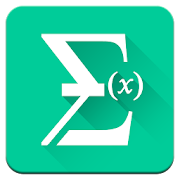## All Math formula helps you practice Grade HS Math - Lumos Educational App StorePrice -FREE

#### DESCRIPTION:

Here is maths formulas pack for all android users. This app has 1000+ math formula and more to come. Now no need to make paper notes to remember mathematics formulas just have this app put all the formulas on your favourite phones. you'll find formulas very simply explained in app with necessary figures will help you to understand very easily. *****Formulas covered in this app***** Algebra - Factoring formulas - Product formulas - Roots formula - Powers formula - Logarithmic formula - Useful equations - Complex number - Binomial theorem Geometry - Cone - Cylinder - Isosc

#### OVERVIEW:

All Math formula is a free educational mobile app By touchfield.It helps students in grades HS practice the following standards HSN.Q.A.1.

This page not only allows students and teachers download All Math formula but also find engaging Sample Questions, Videos, Pins, Worksheets, Books related to the following topics.

1. HSN.Q.A.1 : Use units as a way to understand problems and to guide the solution of multi-step problems; choose and interpret units consistently in formulas; choose and interpret the scale and the origin in graphs and data displays..

HS

#### STANDARDS:

HSN.Q.A.1

Software Version: 1.6

Category: Education

### RELATED APPS

Rate this App?
0

0 Ratings & 0 Reviews

5
0
0
4
0
0
3
0
0
2
0
0
1
0
0EdSearch WebSearch